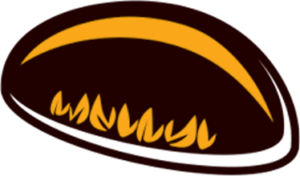# Numeracy

ᐊᑖᓂ, ᐱᓕᕆᐊᒃᓴᓂᒃ ᓇᓂᓯᓂᐊᖅᐳᓯ ᐃᓱᒻᒥᕈᑎᒃᓴᔅᓯᓐᓂᒡᓗ ᐱᓕᕆᐊᖑᔪᓐᓇᖅᑐᓂᒃ ᐃᓕᓐᓂᐊᖅᑎᓄᑦ ᐊᖏᕐᕋᒥ ᐃᓕᓐᓂᐊᕈᓐᓇᖁᓪᓗᒋᑦ.

ᑖᒃᑯᐊ ᐱᓕᕆᔾᔪᑏᑦ JumpMath-ᒦᖖᒑᖅᑐᑦ. ᕿᓂᒃᑲᓐᓂᕈᕕᑦ ᓈᓴᐅᓯᕆᔾᔪᑎᒃᓴᓂᒃ, ᐅᕗᖓ ᖃᐅᔨᒋᐊᕆᑦ https://jumpmath.org/jump/en/.

Below, you will find worksheets and ideas for activities students can try at home to support their learning.

Many of these resources are from JumpMath. If you are looking for additional numeracy resources, visit https://jumpmath.org/jump/en/

Kindergarten Worksheets – Number Sense

### Kindergarten

Kindergarten Worksheets – Essential Lessons (Part 1)

### Kindergarten

Kindergarten Worksheets – Essential Lessons (Part 2)

### Kindergarten

Kindergarten Worksheets – Patterns and Algebra (Part 1)

### Kindergarten

Kindergarten Worksheets – Patterns and Algebra (Part 2)

### Kindergarten

Kindergarten Worksheets – Patterns and Algebra (Part 3)

### Kindergarten

Nunavut Math 1

Grade 1 – Complete Nunavut Math

Worksheets – Geometry

Worksheets – Number Sense I

Worksheets – Number Sense II

Worksheets – Math WorkbookNunavut Math 2

Worksheets – Geometry

Grade 2 – Complete Nunavut Math

Worksheets – Number Sense I (Part 1)

Worksheets – Number Sense I (Part 2)

Worksheets – Number Sense I (Part 3)

Worksheets – Number Sense I (Part 4)

Worksheets – Number Sense II (Part 1)

Worksheets – Number Sense II (Part 2)

Worksheets – Number Sense II (Part 3)

Nunavut Math 3

Worksheets – Number Sense I (Part 1)

Worksheets – Number Sense I (Part 2)

Worksheets – Number Sense I (Part 3)

Worksheets – Number Sense I (Part 4)

Worksheets – Number Sense II

Worksheets – Number Sense III

Worksheets – Number Sense II

Worksheets – Patterns and Algebra

Worksheets – Patterns and Algebra I

Worksheets – Patterns and Algebra II

Worksheets – Number Sense

Worksheets – Geometry

Worksheets – Number Sense

Worksheets – Patterns and Algebra

Worksheets – Geometry

Worksheets – Probability and Data Management

Essential Lessons (Part 1)

Essential Lessons (Part 2)

Worksheet – Geometry

Worksheets – Measurement

Worksheets – Essential Lessons (Part 1)

Worksheets – Essential Lessons (Part 2)

Worksheets – Essential Lessons

Math Outcomes with Evaluation Rubric Updated 2015Math Review Workbook

Math Skills Review Worksheets

Money Math (Part 1)

Money Math (Part 2)

Kitchen Math

Home Math

Activity – Taxman

Activity – Painted Cube

Activity – 36 Fences

Simply Math

Review – Fractions Challenge

### Review

Review – Addition with Big Numbers Challenge

### Review

Review – Writing Numbers Challenge